## NCERT Solutions for Class 11 Chemistry Chapter 3 Classification of Elements and Periodicity in Properties

These Solutions are part of NCERT Solutions for Class 11 Chemistry. Here we have given NCERT Solutions for Class 11 Chemistry Chapter 3 Classification of Elements and Periodicity in Properties.

Question 1.
What is the basic theme of organisation in periodic table ?
The basic theme of organisation in periodic table is to group the elements with same characteristics (physical and chemical) together so that it may become rather easy to follow these characteristics. Since these mainly depend upon the valence shell electronic configurations, the elements placed in a group have therefore same valence shell configuration of their atoms. In fact, the valence shell electronic configuration of the elements placed in a group gets repeated after definite gaps of atomic numbers (8, 8, 18, 18, 32).

Question 2.
Which important property did Mendeleev use to classify the elements in periodic table and did he stick to that ?
The property used by Mendeleev is known as Mendeleev’s Periodic Law. According to the law,

Physical and chemical properties of the elements are a periodic function of their mass numbers.

Mendeleev did stick to it and classified elements into groups and periods. This classification was perhaps the first systematic way in order to study the characteristics of the elements. No doubt, later on many defects were pointed out and scientists challenged the basis of the classification.

Question 3.
What is the basic difference in approach between Mendeleev’s periodic law and Modern periodic law ?
The atomic mass of the elements is the basis of periodicity according to Mendeleev’s periodic law. On the other hand, the atomic number of the elements constitutes the same according to Modern periodic law. For more details,

• Modern Periodic Law

Dmitri Mendeleev, a Russian Chemist, was the first to make a very significant contribution in the formation of the periodic table. He studied the chemical properties of the large number of the elements and in 1869 and came out with a statement that the chemical properties of the elements are a periodic function of their atomic masses. When Mendeleev came to know about the work of Lother Meyer, he modified his statement and gave a law called Mendeleev-Lother Meyer Periodic Law or simply Mendeleev’s Periodic Law.
Modern Periodic Law
It states that In the year 1913, an English physicist, Henry Moseley, a young physicist from England, studied the frequencies of the X-rays which were emitted when certain metals were bombarded with high speed electrons. He found that in all the cases, the square root of the frequency (Vv) was directly proportional to the atomic number of the atom of the metal. Upon investigation, he further stated that there was no co-relation between the frequency and the atomic mass.

Actually, when Moseley plotted a graph between and atomic number of the different metals, a straight line was obtained. But it was not the case when a graph was plotted between (4v) and atomic mass of the metals. These studies led Moseley to believe that atomic number and not the atomic mass is the fundamental property of an element. Therefore, atomic numbers must form the basis of the classification of the elements in the periodic table. In the light of the above observations. Moseley gave the modern periodic law which states that

Question 4.
On the basis of quantum numbers, justify that the sixth period of the periodic table should have 32 elements.
The sixth period corresponds to sixth shell. The sub-shells present in this shell are 6s, 4f, 5p and 6d. The maximum number of electrons which can be present in these sub-shell are 2 + 14 + 6 + 10 = 32. Since the number of elements in a period correspond to the number of electrons in the shells, therefore, sixth period should have a maximum of 32 elements.

Question 5.
In terms of period and group where will you locate the element with Z = 114 ?
We know that the gaps of atomic numbers in a group are 8, 8, 18, 18, 32. The element which proceeds the element with Z = 114 in the same group must have atomic number equal to 114 – 32 = 82. This represents lead (Pb) which is present in 6th period and group 14 of the p-block. This means that the element with Z = 114 must also belong to the group 14 of p-block and it must be a member of 7th period.

Question 6.
Write the atomic number of the element present in the third period and seventeenth group of the periodic table.
The element is chlorine (Cl) with atomic number (Z) = 17.

Question 7.
Which element do you think would have been named by :
(i) Lawrence Berkeley laboratory
(ii) Seaborg’s group ?
(i) Lawrencium (Lr) with atomic number (Z) = 103
(ii) Seaborgium (Sg) with atomic number (Z) = 106.

Question 8.
Why do elements in a group have similar physical and chemical properties ?
The elements in a group have the same valence shell electronic configurations of their atoms. However, they differ in the atomic sizes which tend to increase. Therefore, the elements in a group have similar chemical properties while their physical characteristics show a little variation.

Question 9.
What do atomic radius and ionic radius really mean to you ?
For the definition and details of atomic radius,

Variation of Atomic Radius in the Periodic Table

We shall discuss the variation or change of the atomic radius along the period from left to the right and also down the group.
Variation in a period. Along a period, the atomic radii of the elements generally decrease from left to the right.
The atomic radii (metallic radii for Li, Be and B while covalent radii for the remaining elements) of the elements present in the second period are given :

The trend in atomic radii have also been shown in the figure 3.5. From the values, it is clear that the alkali metal (Li) placed on the extreme left has the maximum atomic radius while the halogen (F) on the extreme right has the minimum value.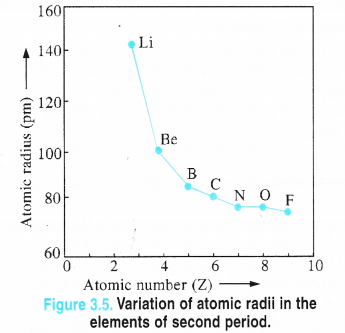Explanation. In moving from left to the right in a period, the nuclear charge gradually increases by one unit and at the same time one electron is also being added in the electron shell. Due to increased nuclear charge from left to the right, the electrons are also getting attracted more and more towards the nucleus. Consequently, the atomic size is expected to decrease as shown in case of the elements of second period.

Ionic compounds are crystalline solids and the ionic radii are related to the ions present in them. The ions formed by the loss of one or more electrons from the neutral atom is known as cation (positive ion) while the one formed by the gain of electrons by the neutral atom is called anion (negative ion). Ionic radius may be defined as :

the effective distance from the centre of the nucleus of the ion upto which it exerts its influence on the electron cloud.

Since the electron cloud may extend itself to a very large distance from the nucleus it may not be possible to determine the ionic radius experimentally. However, some theoretical methods have been given to calculate the radius of the ion and the values as given by Pauling are most acceptable.

But it is quite easy to determine the inter-nuclear distance between two oppositely charged ions (say Na+ and Cl ions) in the crystal lattice by X-ray studies. If the absolute radius of one of them is known, that of the other can be obtained by subtracting the same from the inter-nuclear distance.

Similarly for the details of ionic radius,  In a group, the atomic radii increase downwards.

For example, in sodium chloride crystals, the inter-nuclear distance is 276 pm and the radius of the Na+ ion is 95 pm (Pauling method). Therefore, the radius of CL ion is 276 – 95 = 181 pm.

It may be noted that the value of the ionic radius is linked with the atomic radius and it varies accordingly. Thus, the ionic radius always increases down the group and decreases along the period provided the ions involved have the same magnitude of charge.

This is on account of the increase in the number of electron shells and also due to increase in the magnitude of shielding or screening effect. In a period, the trend is the reverse.

The atomic radii decrease from left to the right because the electrons are filled in the same shell and no new shell is formed. The ionic radii of the elements also follow the same trend.

The atomic radii of the elements influence the other periodic properties such as ionisation enthalpy, electron gain enthalpy and electronegativity.

Question 10.
How do atomic radii vary in a period and in a group ? How do you explain the variation ?
Variation in a group. The atomic radii of the elements in every group of the periodic table increase as we move downwards. Covalent Radii of the alkali metals present in group 1 are given. Since all of them are metallic in nature, their metallic radii have also been given for reference.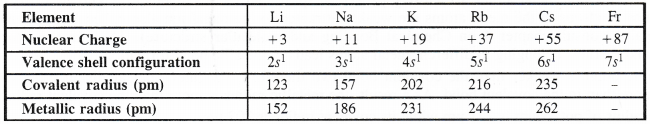The trend in the atomic radii are also shown in the figure 3.6. From the values, it is quite clear that the atomic radius of lithium (Li) is the minimum while that of cesium (Cs) is the maximum. The value of the last element francium (Fr) is not known since being as radioactive in nature, it is unstable.

Explanation. On moving down a group, there is an increase in the principal quantum number and thus, increase in the number of electron shells. Therefore, the atomic size is expected to increase. But at the same time, there is an increase in the atomic number or nuclear charge also. As a result, the atomic size must decrease. However, the effect of increase in the electron shells is more pronounced than the effect of increase in nuclear charge. Consequently, the atomic size or atomic radius increases down a group. This is well supported by the values given in Table 3.5 for the alkali metals.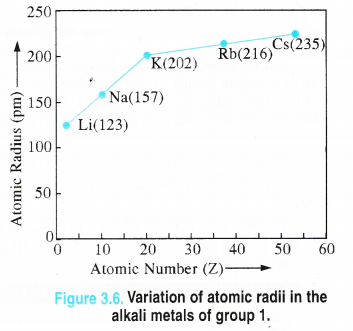Question 11.
What do you understand by isoelectronic species ? Name a species that will be isoelectronic with each of the following atoms or ions.
(i) F (ii) Ar (in) Mg2+ (iv) Rb+.
Isoelectronic species are those species (atoms/ions) which have same number of electrons (iso-same ; electronic- electrons). The isoelectronic species are listed :
(i) Na+
(ii) K+
(iii) Na+
(iv) Sr2+.

Question 12.
Consider the following species :
N3-, O2-, F, Na+, Mg2+, Al3+ .
(a) What is common in them ?
(b) Arrange them in order of increasing radii.
(a) All of them are isoelectronic in nature and have 10 electrons each.

(b)
In isoelectronic species, greater the nuclear charge, lesser will be the atomic or ionic radius. Based upon that, the increasing order of atomic radii are :
Al3+ < Mg2+ < Na+ < F < O2- < N3-.

Question 13.
Explain why are cations smaller and anions larger in radii than their parent atoms.
Cations (positive ions) are formed by the loss of electrons from the parent atoms. Therefore, they have smaller radii than the parent elements. On the other hand, anions are formed when the parent atoms take up one or more electrons. They have therefore, bigger radii than the parent atoms. For illustration,

Question 14.
What is the significance of the terms— ‘isolated gaseous atom’ and ‘ground state’ while defining ionization enthalpy and electron gain enthalpy ?
(a) Significance of term ‘isolated gaseous atom’ : The three states of matter as we know differ in the interparticle spaces. These are the maximum in the solid state while least in the gaseous state.

The atoms in the gaseous state are far separated in the sense that they do not have any mutual attractive and repulsive interactions. These are therefore, regarded as isolated atoms.

When an atom in the gaseous state is isolated, its electron releasing tendency and electron accepting tendency are both absolute in nature.

It means that their values of ionisation enthalpy and of electron gain enthalpy are not influenced by the presence of the other atoms. It is not possible to express these when the atoms are in the liquid or solid state due to the presence of inter atomic forces.

(b) Significance of ground state : The ground state means that in a particular atom, the electrons associated are in the lowest energy state i.e.. they neither lose nor gain electrons. This represents the normal energy state of an atom. Both ionisation enthalpy and electron gain enthalpy are generally expressed with respect to the ground state of an atom only.

Question 15.
The energy of an electron in the ground state of the hydrogen atom is – 2.18 × 10-18 J. Calculate the ionisation enthalpy of atomic hydrogen in terms of J mol-1.
The ionisation enthalpy is for 1 mole atoms. Therefore, ground state energy of one mole atoms may be expressed as:
E(groun state) = (-2.18 × 10-18J) × (6.022 × lO23 mol-1) = – 1.312 × 106Jmol-1
Ionisation enthalpy = Ex – Egroun state
= 0 – (-1.312 × 106 J mol-1) = 1-312 × 106 J mol-1

Question 16.
Among the second period elements, the actual ionization enthalpies are in the order : Li<B<Be<C<O<N<F< Ne.
Explain why
(i) Be has higher ∆iH1 than B ?
(ii) O has lower ∆i8H1 than N and F ?
(i)We know that the cations or positive ions are formed when the neutral atoms (generally metal atoms) lose electrons. They can do so only in the gaseous state since they are isolated and interatomic forces are the minimum. Now energy is needed to overcome the force of attraction between the nucleus and electrons so that the latter may be released. This is known as ionization enthalpy. It may be defined as :
The minimum amount of energy which is needed to remove the most loosely bound electron from a neutral isolated gaseous atom in its ground state to form a cation also in the gaseous state.
Atom (g) → Positive ion (g) + e ; IE (∆iH1)
(Cation)
Na (g) → Na+ (g) + e ; IE (∆iH1)
The energy required can also be expressed in the form of ionization potential which is the minimum amount of potential needed to remove the most loosly held electron from an isolated neutral gaseous atom in its ground state.

(ii)
iH1 values of the there elements present in second period are given :
N(1402 kJ mol-1) ; O(1314 kJ mol-1) ; F(1681 kJ mol-1).
• ∆iH1 of O is expected to more than that of N but is actually lesser because the electronic configuration of N is more symmetrical as well as stable in comparison to O. For electronic configuration.
• ∆iH1 of O is less than that of F because the ionization enthalpy in general increases along a period.

Question 17.
iH1 value of Mg is more as compared to that of Na while its ∆iH2 value is less. Explain.
The electronic configuration of the two elements and their first and second ionization enthalpies are.,given :iH1 value of Mg is more than that of Na due to greater symmetry and smaller size. But ∆iH2 value of Na is higher because Na+ ion has the configuration of noble gas element neon while Mg+ ion does not have a symmetrical configuration.

Question 18.
What are the various factors due to which the ionisation enthalpy of the main group elements tends to decrease down the group ?
Variation down a group. The ionization enthalpies of the elements decrease on moving from top to the bottom in any group. The trend is quite evident from the data given in the table 3.10. The ∆iH1 values of the members belonging to the alkali metals of group 1 have been given separately in the fig 3.10.The decrease in ionization enthalpies down any group is because of the following factors :
(i) There is an increase in the number of the main energy shells (n) in moving from one element to the other.
(ii) There is also an increase in the magnitude of the screening effect due to the gradual increase in the number of inner electrons.
Although the nuclear charge qtlso increases down the group which is likely to result in increased ionization enthalpy but its effect is less pronounced than the two factors listed above. The net result is the decrease in ionization enthalpies in a group in the periodic table.

Question 19.
The first ionisation enthalpy values (kJ mol-1) of group 13 elements are :How would you account for their deviation from the general trend ?
The decrease in ∆iH1 value from B to Al is quite expected because of the bigger size of Al atom. But the element Ga has ten 3d electrons present in the 3d sub-shell which do not screen as much as is done by s and p electrons. Therefore, there is an unexpected increase in the magnitude of effective nuclear charge resulting in increased 3 Classification of Elements and Periodicity in Properties value. The same explanation can be offered in moving from In to T1. The latter has fourteen 4f electrons with very poor shielding effect. This also results in unexpected increase in the effective nuclear charge resulting in inflated ∆iH1 value.

Question 20.
Which of the following pairs of elements would have a more negative electron gain enthalpy ?
(i) O or F
(ii) F or Cl
(i) O or F. The negative electron gain enthalpy of F(∆egH = – 328 kJ mol-1) is more than that of O(∆egH = – 141 kJ mol-1). This is on account of smaller size of the F atom and its greater urge to have a noble gas configuration (only one electron is needed) as compared to oxygen (two electrons are needed).
(ii) F or Cl. The negative electron gain enthalpy of Cl (∆egH = – 349 kJ mol-1) is more than that of F(∆egH = – 328 kJ mol-1). Actually, the size of F atom (atomic radius = 72 pm) is quite small as compared to Cl atom (atomic radius = 99 pm). This results in greater crowding of electrons in small space around the nucleus in case of F and the attraction for outside electron is less as compared to Cl in which the atomic size is large and the crowding of electrons is less.

Question 21.
Would you expect the second electron gain enthalpy of O as positive, more negative or less negative than the first ? Justify your answer.
For oxygen atom ; O(g) + e → 0(g) ; (∆egH) = – 141 kJ mol-1
O(g) e → 02-(g) ; (∆egH) = + 780 kJ mol-1
The first electron gain enthalpy of oxygen is negative because energy is released when a gaseous atom accepts an electron to form monovalent anion. The second electron gain enthalpy is positive because energy is needed to overcome the force of repulsion between monovalent anion and the second incoming electron.

Question 22.
What is basic difference between the terms electron gain enthalpy and electronegativity ?
Difference between electron gain enthalpy and electronegativity.
Both electronegativity and electron gain enthalpy are the electron attracting tendencies of the elements but they differ in many respects. The important points of distinction are listed.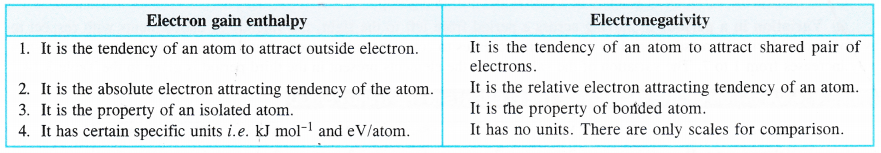Question 23.
How will you react to the statement that the electronegativity of N on Pauling scale is 3-0 in all the nitrogen compounds ?
On Pauling scale, the electronegativity of nitrogen, (3-0) indicates that it is sufficiently electronegative. This is quite expected as well due to its very small size and its requirement to have only three electrons to achieve the noble gas configuration. But it is not correct to say that the electronegativity of nitrogen in all the compounds is 3. It depends upon its state of hydridisation in a particular compound. It may be noted that greater the percentage of ^-character, more will be the electronegativity of the element. Thus, the electronegativity of nitrogen increases in moving from sp3 hybridised orbitals to sp hydridised orbitals i.e., as sp3 < sp2 < sp.

Question 24.
Describe the theory associated with the radius of an atom as it :
(a) gains an electron.
(b) loses an electron.
(a) When an atom gains an electron. It changes into an anion (M + e → M). In
doing so, there is an increase in the size. Actually, there is no change in the atomic number or nuclear charge but there is an increase in the electrons by one. As a result, electrons experience less attraction towards the nucleus and the anionic radius or size increases. For example, the radius of F atom (72 pm) is less than that of F ion (136 pm).

(b) When an atom loses an electron. It changes into a cation (M → M+ + e).
In doing so, there is a decrease in size. In this case also, the nuclear charge is the same but the electrons have decreased by one. As a result, the electrons experience more attraction towards the nucleus and the cationic radius decreases. For example, radius of Na atom (157 pm) is less than that of Na+ ion (95 pm).

Question 25.
What are the major differences between metals and non-metals ?
Metals and non-metals as the names suggest are quite different from one another. They differ in physical as well as chemical characteristics.
Distinction based upon physical properties. The main points of distinction are given :Distinction based upon chemical properties. The main points of distinction are given :Question 26.
Would you expect the ionization enthalpies of two isotopes of the same element to be same or different ? Justify your answer.
The ionization enthalpies are related to the magnitude of nuclear charge as well as the electronic configuration of the elements. Since the isotopes of an element have same nuclear charge as well as electronic configuration, they have the same ionization enthalpies.

Question 27.
Use periodic table to answer the following questions :
(a) Identify the element with five electrons in the outer sub-shell.
(b) Identify the element that would tend to lose two electrons.
(c) Identify the element that would tend to gain two electrons.
(a) Element belongs to nitrogen family (group 15) e.g. nitrogen
(b) Eletnent belongs to alkaline earth family (group 2) e.g. magnesium
(c) Element belongs to oxygen family (group 16) e.g. oxygen

Question 28.
The increasing order of reactivity among group 1 elements is Li < Na < K < Rb < Cs whereas among the group 17 elements, it is F > Cl > Br > I. Explain.
In group 1 elements (alkali metals) the reactivity of the metals is mainly due to the electron releasing tendency of their atoms, which is related to ionization enthalpy. Since ionization enthalpy decreases down the group, the reactivity of alkali metals increases.
In group 17 elements (halogens-non-metals), the reactivity is linked with electron accepting tendency of the members of the family. It is linked with electronegativity and electron gain enthalpy. Since both of them decrease down the group, the reactivity therefore decreases.
Thus, we conclude that the order of reactivity in two cases is different since in one case it is due to electron releasing tendency and it is because of electron accepting tendency in the other case.

Question 29.
Write the general electronic configuration of s, p, d and /block elements.
s-Block elements : ns1-2 (n varies from 2 to 7)
p-Block elements : ns2 np1-6 (n varies from 2 to 7)
d-Block elements : (n – 1) d1-10 ns1-2 (n varies from 4 to 7)
f-Block elements : (n – 2)f1-14 (n – 1) d0-1 ns2 (n may have value either 6 or 7)

Question 30.
Assign the position of the elements having outer electronic configuration
(i) ns2p4 for n = 3
(ii) (n – 1 )d2ns2 for n = 4 and
(iii) (n – 2) f7 (n – 1 )d1 ns2 for n = 6 in the periodic table.
(i) The element is present in third period (n = 3) and belongs to group 16(10 + 2 + 4 = 16).
The element is sulphur : [1s2 2s2 2p6 3s2 3p4].
(ii) The element is present in fourth period (.n = 4) and belongs to group 4 (2 + 2 = 4).
The element is titanium : [Ne] 3d2 4s2.
(iii) The element is present in sixth period (n = 6) and belongs to group 3.
The element is gadolinium (Z = 64): [Xe] 4f7 5d1 6s2.

Question 31.
The first (∆iH1) and the second (∆iH2) ionization enthalpies (in kJ mol-1) and the (∆egH) electron gain enthalpy (in kJ mol1) of a few elements are given below :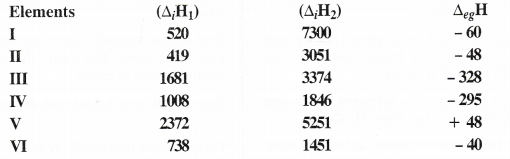Which of the above elements is likely to be :
(a) the least reactive element.
(b) the most reactive metal.
(c) the most reactive non-metal.
(d) the least reactive non-metal.
(e) the metal which can form a stable binary halide of the formula MX2 (X = halogen)
(f) the metal which can form a predominantly stable covalent halide of the formula MX (X = halogen) ?
The nature and reactivity of the elements are guided by ionisation enthalpies and electron gain enthalpies. Based upon
the available data :
(a) The least reactive element is ‘V’. It has very high ∆iH (2372 kJ mol-1) and ∆egH is (+ 48 kJ mol-1) which indicate that it is a noble gas element. The value of ∆egH suggests it to be helium (He).

(b) The most reactive metal is ‘II’. It has a very low ∆iH equal to 419 kJ mol-1 and its negative ∆eg value is also quite small (- 48 kJ mol-1). These values correspond to the alkali metal potassium (K).
In general, alkali metals are the most reactive metals in the periodic table.

(c) The most reactive non-metal is ‘III’. Since the element has very high ionization enthalpies (∆iH1 as well as ∆iH2) and very high negative electron gain enthalpy, it is expected to be a highly reactive non-metal. The values correspond to non-metal fluorine (F).

(d) The least reactive non-metal is ‘IV’. The character is revealed by the moderate values of ∆iH1 and ∆iH2. The value of electron gain enthalpy indicates that the element is iodine (I).

(e) The metal forming stable binary halide (MX2) is ‘VI’. The formula MX2 suggests that the metal belongs to the family of alkaline earth (group 2). The ∆iH1 and ∆iH2 values suggest it to be Mg (However, ∆egH does not agree).

(f) The metal which can form a predominantly stable covalent halide of the formula. MX (halogen) is ‘I’. The metal is monovalent in nature and data suggests it to be lithium (Li) because ∆iH1 ] value is very small as compared to ∆iH2 value. In fact, in alkali metal lithium (Li), the monovalent cation (Li+) has the electronic configuration of the noble gas helium (He) The halide is LiX. The covalent character of LiX is due to the reason that the electron cloud of Li+ ion attracts the electron cloud of X-ion towards itself. As a result, the halide is of covalent nature.

Question 32.
Predict the formulas of the stable binary compounds that would be formed by the combination of the following
pairs of elements :
(a) Lithium and oxygen
(b) Magnesium and nitrogen
(c) Aluminium and iodine
(d) Silicon and oxy gen
(e) Phosphorus and fluorine
(f) Element 71 and fluorine